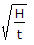# Civil Engineering - Soil Mechanics and Foundation Engineering

### Exercise :: Soil Mechanics and Foundation Engineering - Section 5

6.

The plasticity of fine soils may be assessed by means of

 A. dry strength test B. toughness test C. dilatancy test D. all of these.

Explanation:

No answer description available for this question. Let us discuss.

7.

The density of soil can be increased

 A. by reducing the space occupied by air B. by elastic compression of soil grains C. by expelling water from pores D. All the above.

Explanation:

No answer description available for this question. Let us discuss.

8.

The length/diameter ratio of cylindrical specimens used in triaxial test, is generally

 A. 1 B. 1.5 C. 2 D. 2.5 E. 3

Explanation:

No answer description available for this question. Let us discuss.

9.

Pick up the correct statement from the following:

 A. The object of classifying soils is to arrange them into groups according to their properties and behaviour B. A soil classification system is meant to provide an accepted and systematic method of describing the various types of soils eliminating personal factors C. The first category of soil classification is based on grain size of the soil D. The second category of soil classification is based on fine as well as coarse grains E. All the above.

Explanation:

No answer description available for this question. Let us discuss.

10.

A suspended particle falls through a height H cm in water in t minutes. If the viscosity of water is η and specific gravity of the particle is G, the diameter of the particle is (where M is a constant factor)

 A. 103MB. 104MC. 105MD. 102M